Last Updated On

In today’s article on Electricity, I will be explaining how to identify if bulbs are arranged in series or parallel before discussing their respective advantages and disadvantages with the aid of a question.

Previously, we have discussed the arrangement of wires in a light bulb and its effects.

In addition to understanding that concept, it is also important for students to be able to identify the different arrangements of bulbs in an electrical circuit – series and parallel.

After which, they have to understand that arranging the bulbs in a certain arrangement has its advantages and disadvantages.

## Question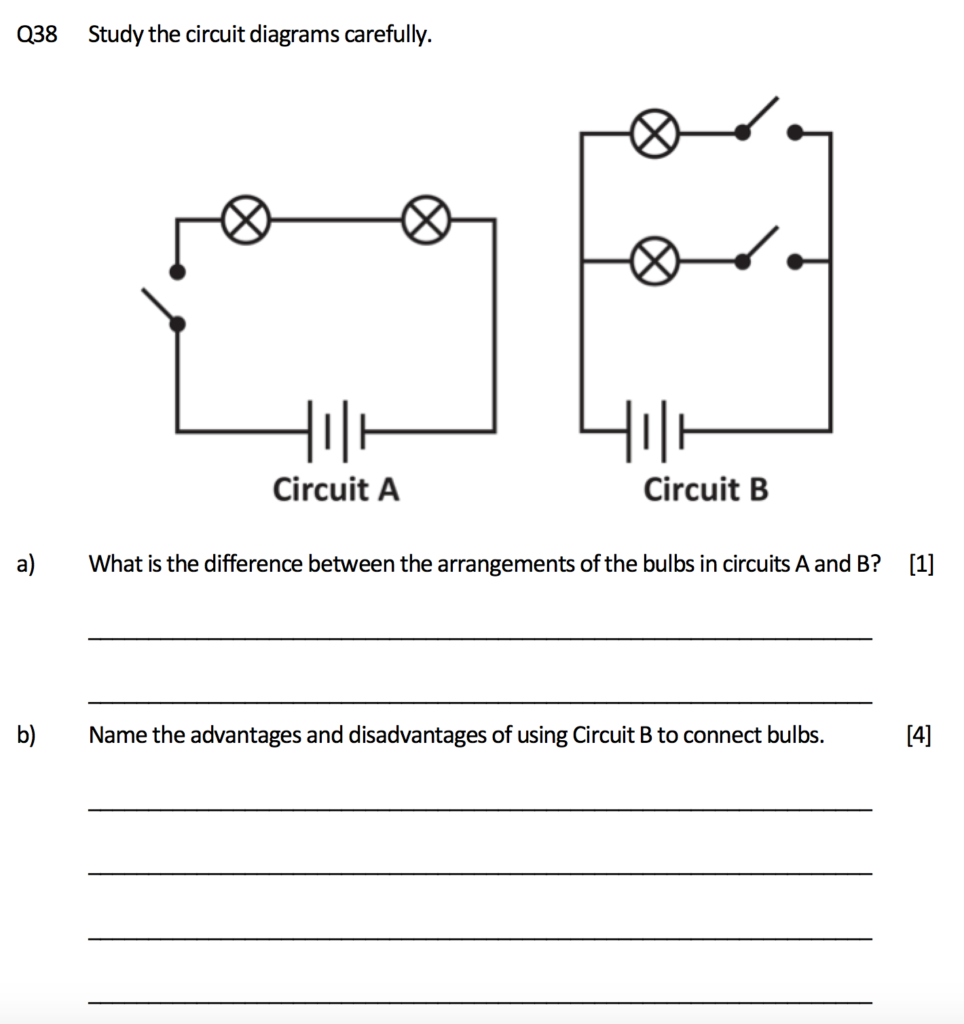### Diagrams of Circuit A and Circuit B

We can see from the diagrams that both Circuit A and Circuit B have 2 batteries and 2 bulbs each.

The only difference (other than the number of switches) is the position of the bulbs in the circuit. When there are two bulbs in a circuit, they can either be arranged in series or in parallel.

However, many students often have misconceptions about the definition of bulbs in “series” and “parallel”. They tend to associate bulbs arranged in series to be bulbs placed next to each other and bulbs in parallel to be one bulb placed on top/below of another bulb, which is inaccurate.

When the bulbs in a circuit are arranged in series, there is only one pathway that electricity can flow through. When bulbs are arranged in parallel, there is more than one pathway in the circuit that electricity can flow through.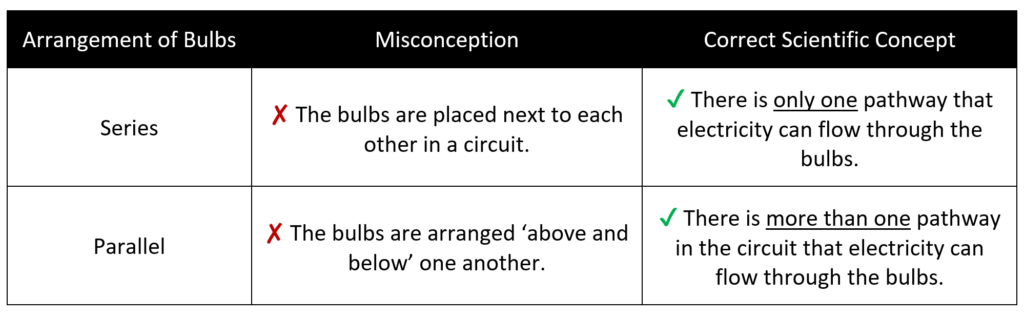Therefore, in order to identify the arrangements of bulbs in a circuit correctly, students should identify the number of pathways that electricity is able to flow through in a circuit.

Circuit A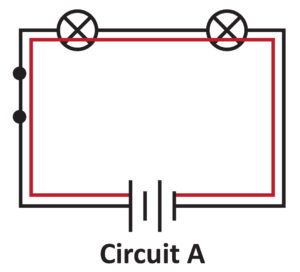In the diagram above, I have traced in red how electricity flows from one end to the other end of the battery for Circuit A. We can see that there is only one pathway that electricity can flow through the bulbs in Circuit A. Thus, the bulbs in Circuit A are arranged in series.

Circuit B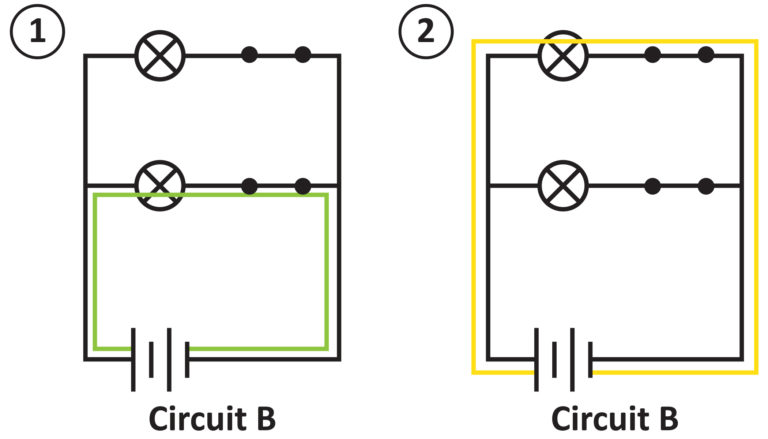In the two diagrams above, I have traced in green and yellow the two possible pathways that electricity can flow through from one end of the battery to the other. With these diagrams, it is clear that there is more than one possible pathway that electricity can flow through the bulbs. Thus, the bulbs in Circuit B are arranged in parallel.

## Answer For Part (a)

(a) What is the difference between the arrangements of the bulbs in circuits A and B?

The bulbs in Circuit A are arranged in series while the bulbs in Circuit B are arranged in parallel.

### Advantages and Disadvantages of Arranging Bulbs in Series or Parallel

Now that we have gained a better understanding of what it means to arrange bulbs in series and in parallel, let us discuss the advantages and disadvantages of these two arrangements. There are four points of comparison between the series arrangement and the parallel arrangement of bulbs:

1. Brightness of the bulb
2. Lifespan of batteries
3. Independent control of bulbs
4. Whether the other bulbs in the circuit will still light up when one bulb fuses

### Comparison 1: Brightness of the bulb

The number of batteries in a circuit determines the amount of electricity flowing through each pathway. Additionally, the brightness of the bulb corresponds to the amount of electricity it receives. With the above in mind, let us now work out the brightness of the bulbs together. Note: 1 battery represents 1 unit of electricity.

Series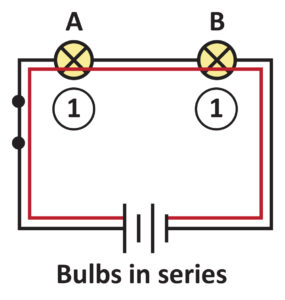In the circuit above, there are two batteries. This means that there are 2 units of electricity flowing through the red pathway. As electricity passes through both Bulbs A and B, the two bulbs share the 2 units of electricity equally. 2 units of electricity ÷ 2 bulbs → each bulb receives 1 unit of electricity Since the brightness of the bulb corresponds to the amount of electricity it receives, each bulb in this series circuit has a brightness of 1 unit.

Parallel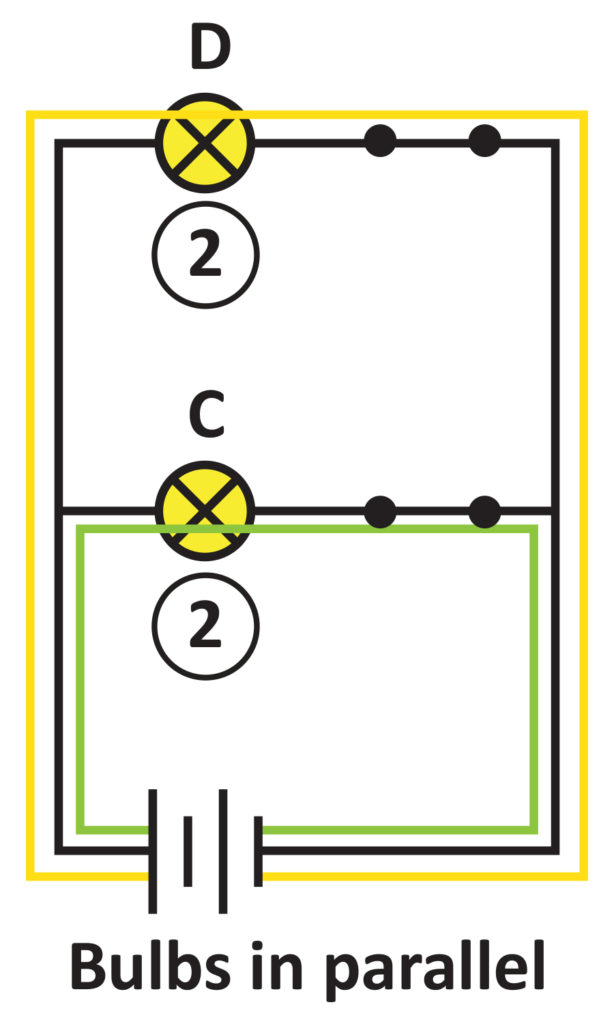Recall that the number of batteries in a circuit determines the amount of electricity flowing through each pathway.]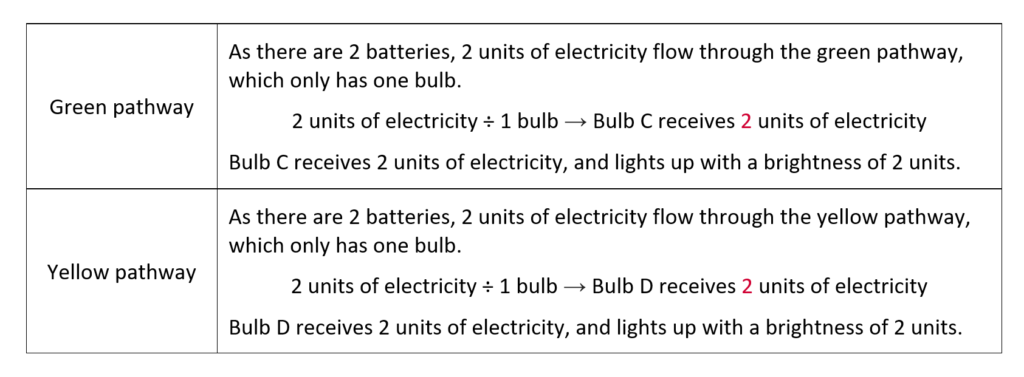Comparing the brightness of the bulbs

The bulbs in the series circuit have a brightness of 1 unit, while the bulbs in the parallel circuit have a brightness of 2 units.

Therefore, we can see that if all other variables were kept constant, bulbs arranged in parallel are brighter than bulbs arranged in series.

### Comparison 2: Lifespan of batteries

Series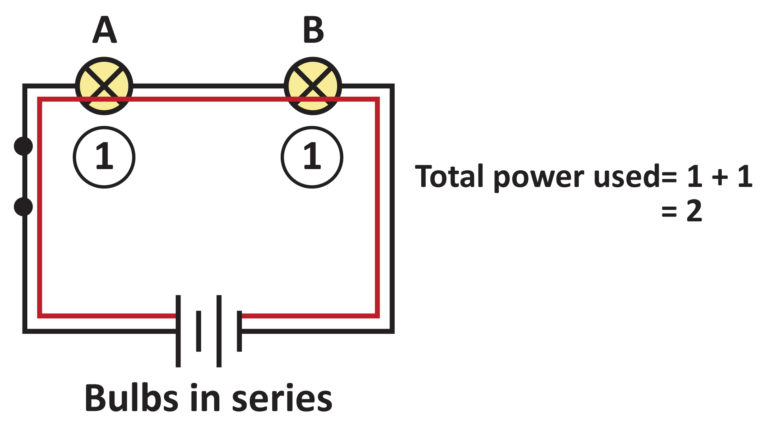Each bulb in the above circuit uses 1 unit of electricity. Therefore, in total, the batteries need to produce 2 units of electricity for the bulbs arranged in series.

Parallel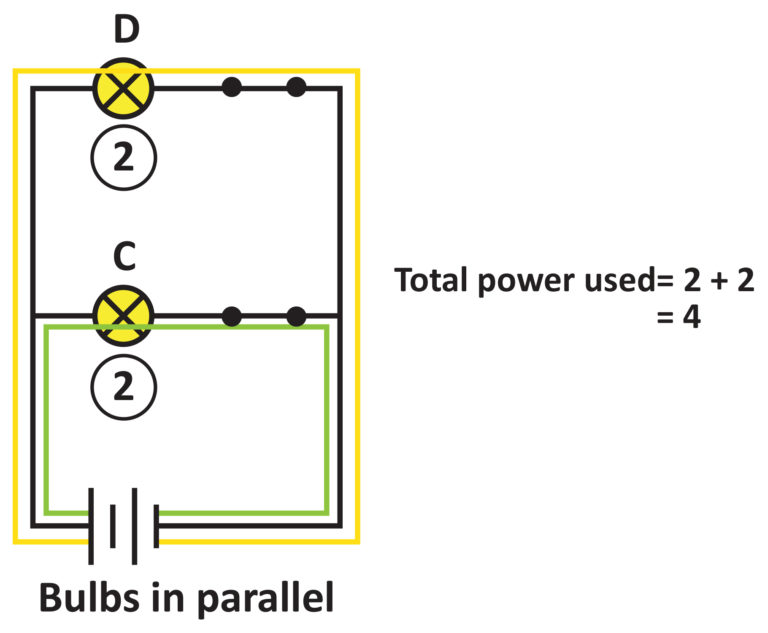Each bulb in the above circuit uses 2 units of electricity. Therefore, in total, the batteries need to produce 4 units of electricity for the bulbs arranged in parallel. Comparing the lifespan of the batteries The batteries in a parallel circuit have to produce more units of electricity than the batteries in a series circuit. Thus, we can infer that the batteries in a circuit with bulbs arranged in parallel will be used up more quickly and have a shorter lifespan.

### Comparison 3: Independent control of bulbs

Series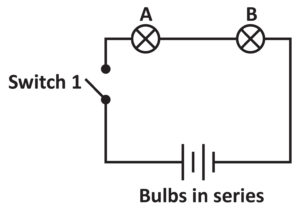When switch 1 is open, there is an open circuit. Electricity is unable to flow through both bulbs A and B, preventing these bulbs from lighting up.

Parallel

Depending on which part of the circuit where switches are installed, the bulbs can be controlled independently.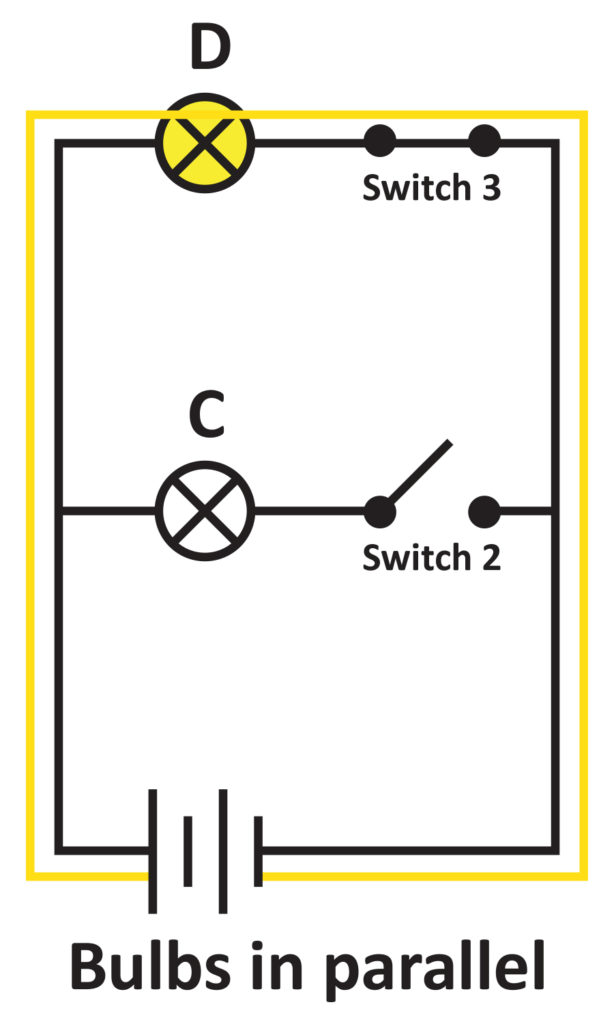In the case of the above circuit, when switch 2 is open, there is an open circuit with Bulb C.

Electricity is unable to flow through Bulb C, preventing Bulb C from lighting up. However, as switch 3 is closed, there is still a closed circuit with Bulb D.  Electricity is able to flow through Bulb D, allowing Bulb D to light up.

Comparing the degree of control

From the above, we can see that the bulbs arranged in parallel can be controlled independently of each other, whereas the bulbs in series will always be switched on or off together.

### Comparison 4: Whether the other bulbs in the circuit will still light up when one bulb fuses

Let me do a quick introduction on what does it mean for a bulb to fuse.

The filament is the part of the bulb that glows when electricity flows through, causing the bulb to light up. When too much electricity flows through the filament, the filament overheats and melts, resulting in a gap.When bulbs have a melted filament, they have fused. Due to the gap in the filament, electricity is unable to flow through the filaments of fused bulbs, preventing them from lighting up. How will one fused bulb affect the other bulbs in the circuits? The outcome depends on how the bulbs are arranged in the circuit.

Series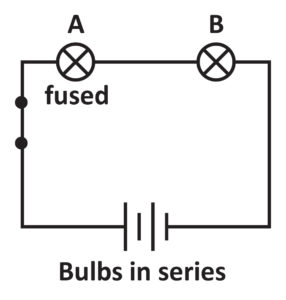When Bulb A is fused, there is an open circuit. Electricity would not be able to flow through Bulb A, and consequently, Bulb B. Thus, Bulb B would not light up.

Parallel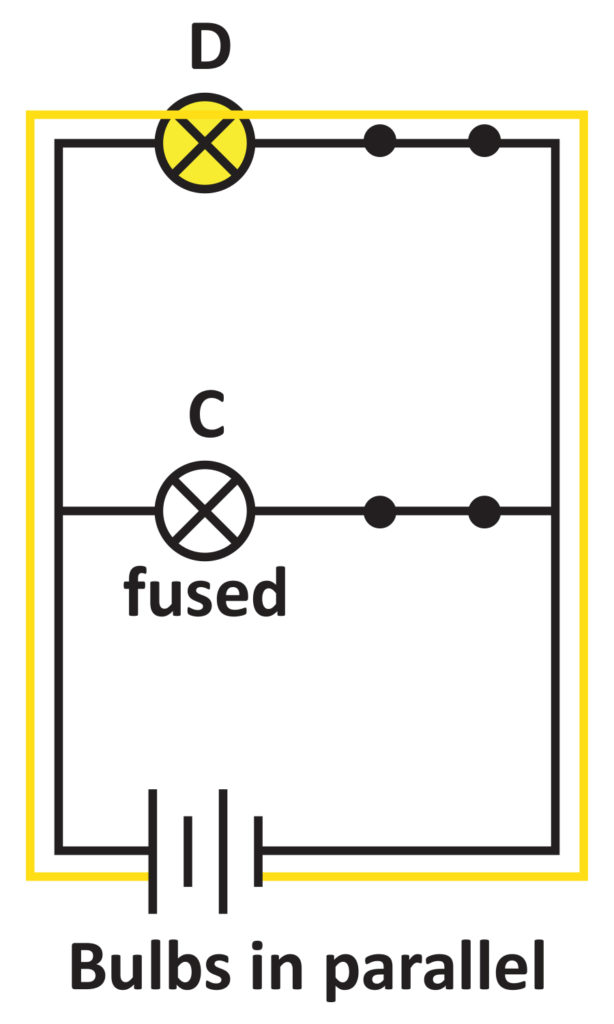When Bulb C is fused, there is still a closed circuit with Bulb D. Electricity would be able to flow through Bulb D, allowing Bulb D to light up.

Comparing the outcome when one of the bulbs fuses

When one of the bulbs in a parallel arrangement fuses, the other bulbs in the circuit are still able to light up. On the other hand, when one of the bulbs in a series arrangement fuses, the other bulbs in the circuit will not light up. With the above analysis, let us now answer part (b). As the bulbs in Circuit B are arranged in parallel, we shall name the advantages and disadvantages of connecting bulbs in parallel.

## Answer For Part (b)

#### (b) Name the advantages and disadvantages of using Circuit B to connect bulbs.

1. The bulbs connected using Circuit B would be brighter, and the bulbs can be controlled independently. [Advantage]
2. Additionally, when one bulb fuses, there would still be a closed circuit with the other bulbs in circuit B. Electricity would still be able to flow through the other bulbs, allowing them to light up. [Advantage]
3. However, the batteries in Circuit B will be used up more quickly. [Disadvantage]

## What Did We Learn Today?

1. When there are two or more bulbs in a circuit, they can be arranged in series or parallel.
2. When bulbs are arranged in series, there is only one pathway that electricity can flow through the bulbs.
3. When bulbs are arranged in parallel, there is more than one possible pathway that electricity can flow through the bulbs.
4. Each arrangement has its advantages and disadvantages.

In the next article, I will be sharing more about conductors and insulators of electricity.If you like our approach behind solving 'Electricity' questions, perhaps you may be interested to check out our upcoming Electrical Circuit Masterclass where we'll dive deep into several answering techniques and practice questions.